# Visual MCMC diagnostics using the bayesplot package

## Introduction

This vignette focuses on MCMC diagnostic plots, in particular on diagnosing divergent transitions and on the n_eff and Rhat statistics that help you determine that the chains have mixed well. Plots of parameter estimates from MCMC draws are covered in the separate vignette Plotting MCMC draws, and graphical posterior predictive model checking is covered in the Graphical posterior predictive checks vignette.

Note that most of these plots can also be browsed interactively using the shinystan package.

### Setup

In addition to bayesplot we’ll load the following packages:

• ggplot2, in case we want to customize the ggplot objects created by bayesplot
• rstan, for fitting the example models used throughout the vignette
library("bayesplot")
library("ggplot2")
library("rstan")      

### Example model

Before we delve into the actual plotting we need to fit a model to have something to work with. In this vignette we’ll use the eight schools example, which is discussed in many places, including Rubin (1981), Gelman et al. (2013), and the RStan Getting Started wiki. This is a simple hierarchical meta-analysis model with data consisting of point estimates y and standard errors sigma from analyses of test prep programs in J=8 schools. Ideally we would have the full data from each of the previous studies, but in this case we only have the these estimates.

schools_dat <- list(
J = 8,
y = c(28,  8, -3,  7, -1,  1, 18, 12),
sigma = c(15, 10, 16, 11,  9, 11, 10, 18)
)

The model is: \begin{align*} y_j &\sim {\rm Normal}(\theta_j, \sigma_j), \quad j = 1,\dots,J \\ \theta_j &\sim {\rm Normal}(\mu, \tau), \quad j = 1, \dots, J \\ \mu &\sim {\rm Normal}(0, 10) \\ \tau &\sim {\rm half-Cauchy}(0, 10), \end{align*} with the normal distribution parameterized by the mean and standard deviation, not the variance or precision. In Stan code:

// Saved in 'schools_mod_cp.stan'
data {
int<lower=0> J;
vector[J] y;
vector<lower=0>[J] sigma;
}
parameters {
real mu;
real<lower=0> tau;
vector[J] theta;
}
model {
mu ~ normal(0, 10);
tau ~ cauchy(0, 10);
theta ~ normal(mu, tau);
y ~ normal(theta, sigma);
}

This parameterization of the model is referred to as the centered parameterization (CP). We’ll also fit the same statistical model but using the so-called non-centered parameterization (NCP), which replaces the vector $$\theta$$ with a vector $$\eta$$ of a priori i.i.d. standard normal parameters and then constructs $$\theta$$ deterministically from $$\eta$$ by scaling by $$\tau$$ and shifting by $$\mu$$: \begin{align*} \theta_j &= \mu + \tau \,\eta_j, \quad j = 1,\dots,J \\ \eta_j &\sim N(0,1), \quad j = 1,\dots,J. \end{align*} The Stan code for this model is:

// Saved in 'schools_mod_ncp.stan'
data {
int<lower=0> J;
vector[J] y;
vector<lower=0>[J] sigma;
}
parameters {
real mu;
real<lower=0> tau;
vector[J] eta;
}
transformed parameters {
vector[J] theta;
theta = mu + tau * eta;
}
model {
mu ~ normal(0, 10);
tau ~ cauchy(0, 10);
eta ~ normal(0, 1); // implies theta ~ normal(mu, tau)
y ~ normal(theta, sigma);
}

The centered and non-centered are two parameterizations of the same statistical model, but they have very different practical implications for MCMC. Using the bayesplot diagnostic plots, we’ll see that, for this data, the NCP is required in order to properly explore the posterior distribution.

To fit both models we first translate the Stan code to C++ and compile it using the stan_model function.

schools_mod_cp <- stan_model("schools_mod_cp.stan")
schools_mod_ncp <- stan_model("schools_mod_ncp.stan")

We then fit the model by calling Stan’s MCMC algorithm using the sampling function (the increased adapt_delta param is to make the sampler a bit more “careful” and avoid false positive divergences),

fit_cp <- sampling(schools_mod_cp, data = schools_dat, seed = 803214053, control = list(adapt_delta = 0.9))
Warning: There were 206 divergent transitions after warmup. See
http://mc-stan.org/misc/warnings.html#divergent-transitions-after-warmup
to find out why this is a problem and how to eliminate them.
Warning: There were 1 chains where the estimated Bayesian Fraction of Missing Information was low. See
http://mc-stan.org/misc/warnings.html#bfmi-low
Warning: Examine the pairs() plot to diagnose sampling problems
Warning: The largest R-hat is 1.09, indicating chains have not mixed.
Running the chains for more iterations may help. See
http://mc-stan.org/misc/warnings.html#r-hat
Warning: Bulk Effective Samples Size (ESS) is too low, indicating posterior means and medians may be unreliable.
Running the chains for more iterations may help. See
http://mc-stan.org/misc/warnings.html#bulk-ess
Warning: Tail Effective Samples Size (ESS) is too low, indicating posterior variances and tail quantiles may be unreliable.
Running the chains for more iterations may help. See
http://mc-stan.org/misc/warnings.html#tail-ess
fit_ncp <- sampling(schools_mod_ncp, data = schools_dat, seed = 457721433, control = list(adapt_delta = 0.9))

and extract a iterations x chains x parameters array of posterior draws with as.array,

# Extract posterior draws for later use
posterior_cp <- as.array(fit_cp)
posterior_ncp <- as.array(fit_ncp)

You may have noticed the warnings about divergent transitions for the centered parametrization fit. Those are serious business and in most cases indicate that something is wrong with the model and the results should not be trusted. For an explanation of these warnings see Divergent transitions after warmup. We’ll have a look at diagnosing the source of the divergences first and then dive into some diagnostics that should be checked even if there are no warnings from the sampler.

## Diagnostics for the No-U-Turn Sampler

The No-U-Turn Sampler (NUTS, Hoffman and Gelman, 2014) is the variant of Hamiltonian Monte Carlo (HMC) used by Stan and the various R packages that depend on Stan for fitting Bayesian models. The bayesplot package has special functions for visualizing some of the unique diagnostics permitted by HMC, and NUTS in particular. See Betancourt (2017), Betancourt and Girolami (2013), and Stan Development Team (2017) for more details on the concepts.

Documentation:

The special bayesplot functions for NUTS diagnostics are

available_mcmc(pattern = "_nuts_")
bayesplot MCMC module:
(matching pattern '_nuts_')
mcmc_nuts_acceptance
mcmc_nuts_divergence
mcmc_nuts_energy
mcmc_nuts_stepsize
mcmc_nuts_treedepth

Those functions require more information than simply the posterior draws, in particular the log of the posterior density for each draw and some NUTS-specific diagnostic values may be needed. The bayesplot package provides generic functions log_posterior and nuts_params for extracting this information from fitted model objects. Currently methods are provided for models fit using the rstan, rstanarm and brms packages, although it is not difficult to define additional methods for the objects returned by other R packages. For the Stan models we fit above we can use the log_posterior and nuts_params methods for stanfit objects:

lp_cp <- log_posterior(fit_cp)
head(lp_cp)
  Chain Iteration     Value
1     1         1 -15.76023
2     1         2 -21.53741
3     1         3 -20.17119
4     1         4 -18.52812
5     1         5 -19.10313
6     1         6 -17.19231
np_cp <- nuts_params(fit_cp)
head(np_cp)
  Chain Iteration     Parameter     Value
1     1         1 accept_stat__ 0.8986435
2     1         2 accept_stat__ 0.9864082
3     1         3 accept_stat__ 0.9797520
4     1         4 accept_stat__ 0.9971116
5     1         5 accept_stat__ 0.9975943
6     1         6 accept_stat__ 0.9915813
# for the second model
lp_ncp <- log_posterior(fit_ncp)
np_ncp <- nuts_params(fit_ncp)

In addition to the NUTS-specific plotting functions, some of the general MCMC plotting functions demonstrated in the Plotting MCMC draws vignette also take optional arguments that can be used to display important HMC/NUTS diagnostic information. We’ll see examples of this in the next section on divergent transitions.

### Divergent transitions

When running the Stan models above, there were warnings about divergent transitions. Here we’ll look at diagnosing the source of divergences through visualizations.

#### mcmc_parcoord

The mcmc_parcoord plot shows one line per iteration, connecting the parameter values at this iteration. This lets you see global patterns in the divergences.

This function works in general without including information about the divergences, but if the optional np argument is used to pass NUTS parameter information, then divergences will be colored in the plot (by default in red).

# not evaluated to reduce vignette size for CRAN
# full version available at mc-stan.org/bayesplot/articles
color_scheme_set("darkgray")
mcmc_parcoord(posterior_cp, np = np_cp)

Here, you may notice that divergences in the centered parameterization happen exclusively when tau, the hierarchical standard deviation, goes near zero and the values of the thetas are essentially fixed. This makes tau immediately suspect. See Gabry et al. (2019) for another example of the parallel coordinates plot.

#### mcmc_pairs

The mcmc_pairs function can also be used to look at multiple parameters at once, but unlike mcmc_parcoord (which works well even when including several dozen parameters) mcmc_pairs is more useful for up to ~8 parameters. It shows univariate histograms and bivariate scatter plots for selected parameters and is especially useful in identifying collinearity between variables (which manifests as narrow bivariate plots) as well as the presence of multiplicative non-identifiabilities (banana-like shapes).

Let’s look at how tau interacts with other variables, using only one of the thetas to keep the plot readable:

# not evaluated to reduce vignette size for CRAN
# full version available at mc-stan.org/bayesplot/articles
mcmc_pairs(posterior_cp, np = np_cp, pars = c("mu","tau","theta"),
off_diag_args = list(size = 0.75))

Note that each bivariate plot is present twice – by default each of those contain half of the chains, so you also get to see if the chains produced similar results (see the documentation for the condition argument for other options). Here, the interaction of tau and theta seems most interesting, as it concentrates the divergences into a tight region.

Further examples of pairs plots and instructions for using the various optional arguments to mcmc_pairs are provided via help("mcmc_pairs").

#### mcmc_scatter

Using the mcmc_scatter function (with optional argument np) we can look at a single bivariate plot to investigate it more closely. For hierarchical models, a good place to start is to plot a “local” parameter (theta[j]) against a “global” scale parameter on which it depends (tau).

We will also use the transformations argument to look at the log of tau, as this is what Stan is doing under the hood for parameters like tau that have a lower bound of zero. That is, even though the draws for tau returned from Stan are all positive, the parameter space that the Markov chains actual explore is unconstrained. Transforming tau is not strictly necessary for the plot (often the plot is still useful without it) but plotting in the unconstrained is often even more informative.

First the plot for the centered parameterization:

# assign to an object so we can reuse later
scatter_theta_cp <- mcmc_scatter(
posterior_cp,
pars = c("theta", "tau"),
transform = list(tau = "log"), # can abbrev. 'transformations'
np = np_cp,
size = 1
)
scatter_theta_cp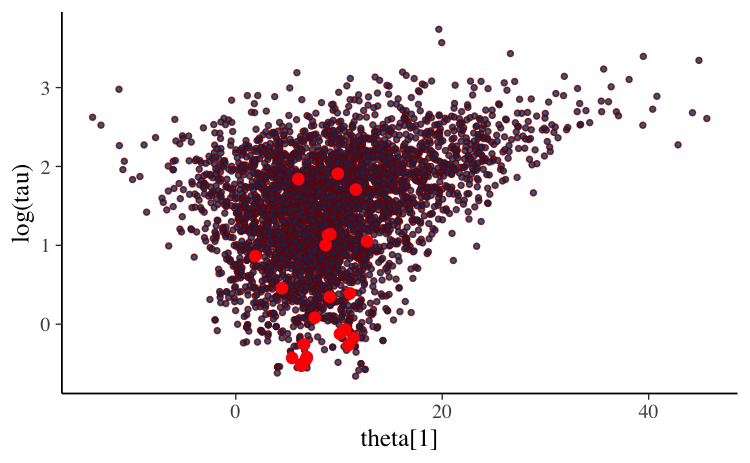The shape of this bivariate distribution resembles a funnel (or tornado). This one in particular is essentially the same as an example referred to as Neal’s funnel (details in the Stan manual) and it is a clear indication that the Markov chains are struggling to explore the tip of the funnel, which is narrower than the rest of the space.

The main problem is that large steps are required to explore the less narrow regions efficiently, but those steps become too large for navigating the narrow region. The required step size is connected to the value of tau. When tau is large it allows for large variation in theta (and requires large steps) while small tau requires small steps in theta.

The non-centered parameterization avoids this by sampling the eta parameter which, unlike theta, is a priori independent of tau. Then theta is computed deterministically from the parameters eta, mu and tau afterwards. Here’s the same plot as above, but with eta from non-centered parameterization instead of theta from the centered parameterization:

scatter_eta_ncp <- mcmc_scatter(
posterior_ncp,
pars = c("eta", "tau"),
transform = list(tau = "log"),
np = np_ncp,
size = 1
)
scatter_eta_ncp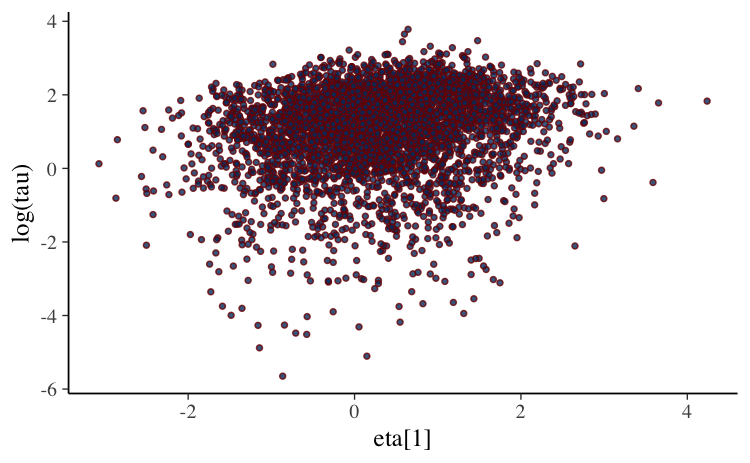We can see that the funnel/tornado shape is replaced by a somewhat Gaussian blob/cloud and the divergences go away. Gabry et al. (2019) has further discussion of this example.

Ultimately we only care about eta insofar as it enables the Markov chains to better explore the posterior, so let’s directly examine how much more exploration was possible after the reparameterization. For the non-centered parameterization we can make the same scatterplot but use the values of theta = mu + eta * tau instead of eta. Below is a side by side comparison with the scatterplot of theta vs log(tau) from the centered parameterization that we made above. We will also force the plots to have the same $$y$$-axis limits, which will make the most important difference much more apparent:

# A function we'll use several times to plot comparisons of the centered
# parameterization (cp) and the non-centered parameterization (ncp). See
# help("bayesplot_grid") for details on the bayesplot_grid function used here.
compare_cp_ncp <- function(cp_plot, ncp_plot, ncol = 2, ...) {
bayesplot_grid(
cp_plot, ncp_plot,
grid_args = list(ncol = ncol),
subtitles = c("Centered parameterization",
"Non-centered parameterization"),
...
)
}

scatter_theta_ncp <- mcmc_scatter(
posterior_ncp,
pars = c("theta", "tau"),
transform = list(tau = "log"),
np = np_ncp,
size = 1
)

compare_cp_ncp(scatter_theta_cp, scatter_theta_ncp, ylim = c(-8, 4))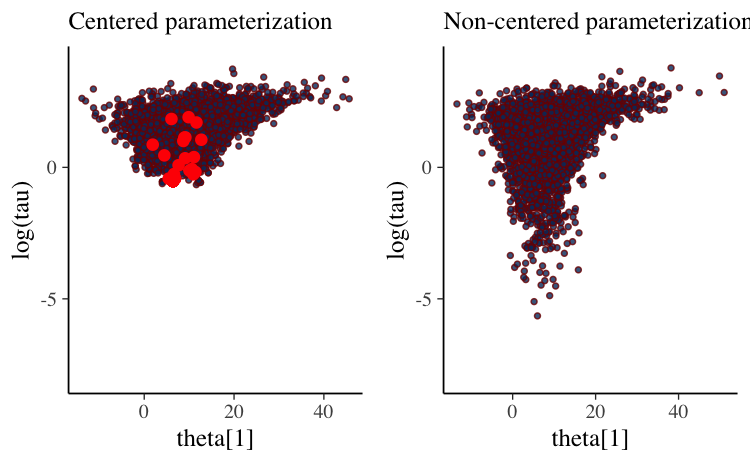Once we transform the eta values into theta values we actually see an even more pronounced funnel/tornado shape than we have with the centered parameterization. But this is precisely what we want! The non-centered parameterization allowed us to obtain draws from the funnel distribution without having to directly navigate the curvature of the funnel. With the centered parameterization the chains never could make it into the neck of funnel and we see a clustering of divergences and no draws in the tail of the distribution.

#### mcmc_trace

Another useful diagnostic plot is the trace plot, which is a time series plot of the Markov chains. That is, a trace plot shows the evolution of parameter vector over the iterations of one or many Markov chains. The np argument to the mcmc_trace function can be used to add a rug plot of the divergences to a trace plot of parameter draws. Typically we can see that at least one of the chains is getting stuck wherever there is a cluster of many red marks.

Here is the trace plot for the tau parameter from the centered parameterization:

color_scheme_set("mix-brightblue-gray")
mcmc_trace(posterior_cp, pars = "tau", np = np_cp) +
xlab("Post-warmup iteration")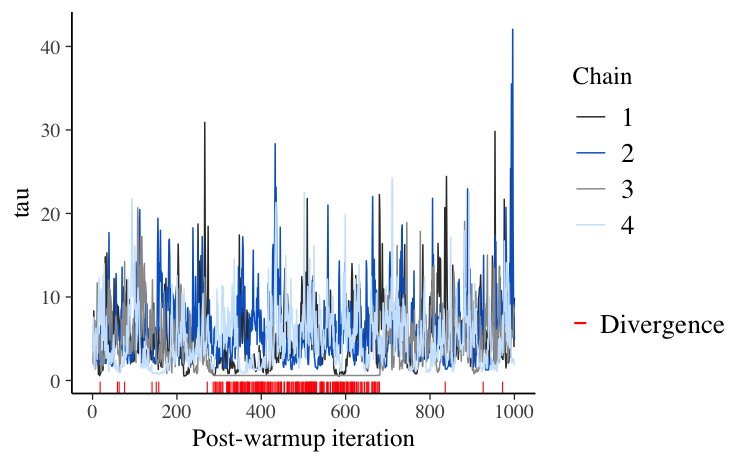The first thing to note is that all chains seem to be exploring the same region of parameter values, which is a good sign. But the plot is too crowded to help us diagnose divergences. We may however zoom in to investigate, using the window argument:

mcmc_trace(posterior_cp, pars = "tau", np = np_cp, window = c(300,500)) +
xlab("Post-warmup iteration")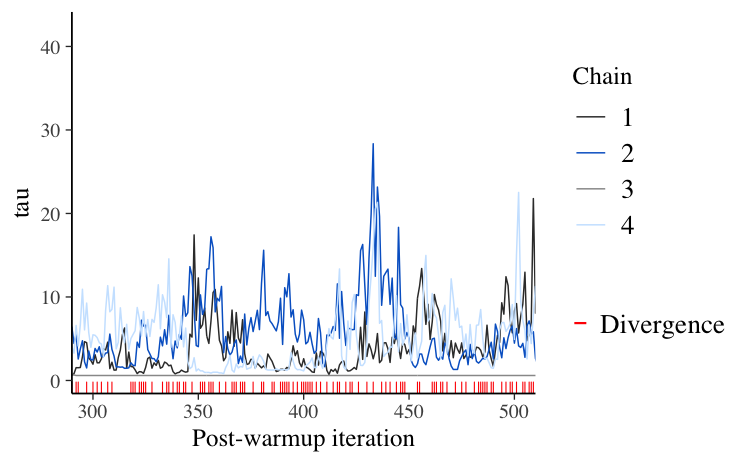What we see here is that chains can get stuck as tau approaches zero and spend substantial time in the same region of the parameter space. This is just another indication that there is problematic geometry at $$\tau \simeq 0$$ – healthy chains jump up and down frequently.

#### mcmc_nuts_divergence

To understand how the divergences interact with the model globally, we can use the mcmc_nuts_divergence function:

color_scheme_set("red")
mcmc_nuts_divergence(np_cp, lp_cp)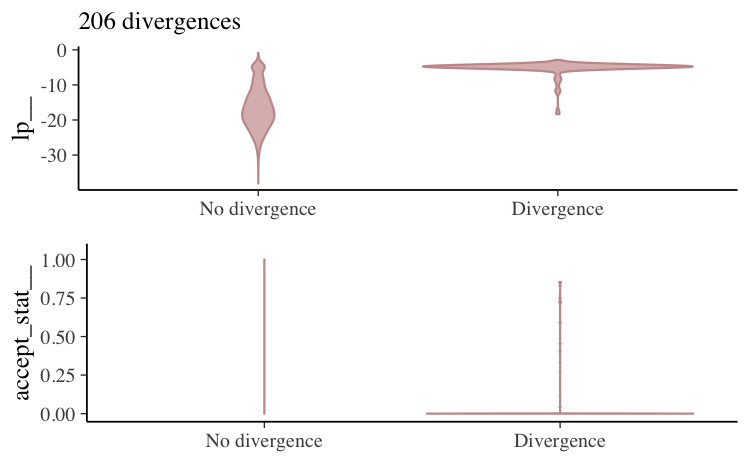In the top panel we see the distribution of the log-posterior when there was no divergence vs the distribution when there was a divergence. Divergences often indicate that some part of the posterior isn’t being explored and the plot confirms that lp|Divergence indeed has lighter tails than lp|No divergence.

The bottom panel shows the same thing but instead of the log-posterior the NUTS acceptance statistic is shown.

Specifying the optional chain argument will overlay the plot just for a particular Markov chain on the plot for all chains combined:

mcmc_nuts_divergence(np_cp, lp_cp, chain = 4)